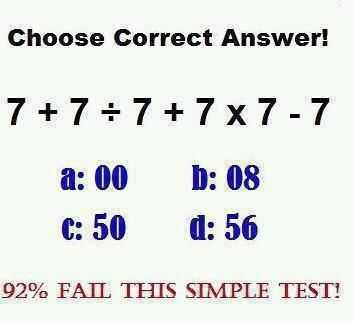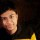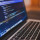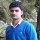Tough Puzzles & Brain Teasers
Puzzles on logic and mathematics that will make you scratch your head.
75 Members
Join this group to post and comment.Ankita Katdare
Computer Science
08 Jan 2019

# 7 + 7 / 7 + 7 * 7 - 7 Puzzle - What is the correct answer?

Choose the correct answer for this equation: 7 + 7 / 7 + 7 * 7 - 7

Is it a: 00 b: 08 c:50 d:56Computer Science
2mos ago

The equation can be rewritten in this way-

[{(7+7)/(7+7)}*(7-7)]

So, according to the BODMAS rule, division has first priority so the numbers in the curve bracket would undergo operation first.

{(7+7)/(7+7)}= 14/14= 1

Now, 1*(7-7)=1*0=0

So, the answer is a) 00.

Correct me if I am wrong..?Ankita Katdare
Computer Science
2mos ago

Let's wait for more people to answer so that we can see if there are any other answers to this.Aswini Vi
Computer Science
2mos ago

Consider the BODMAS rule to solve this problem.

As per the BODMAS rule we need to solve brackets ,but we don't have brackets in question.

Next is division 7/7=1 ,so the equation will get change into 7+1+7*7-7.

Then multiplication 7*7=49 and equation changed to 7+1+49-7.

Now by applying addition to above equation,we will get 57-7.

Finally ,perform subtraction 57-5=50

So the answer is 50Vijay Khatri
Computer Science
2mos ago

The very simple answer:

B: Bracket

O: Of

D: Division

M: Multiplication

S: Subtraction

In this question there is no bracket then we will start from divide:

7+7/7+7*7-7

7+1+7*7-7

After division, we will go for Multiplication:

7+1+49-7

After Multiplication, we will go for Addition:

57-7

After Addition, we will go for subtraction:

50

So 50 is the correct answer, I will go with the Aswini Variganji | CrazyEngineers Even he mentioned wrong in their method 57-5=50Aswini Vi
Computer Science
2mos ago

The final step at subtraction ,I did a mistake .

Actually it was 57-7